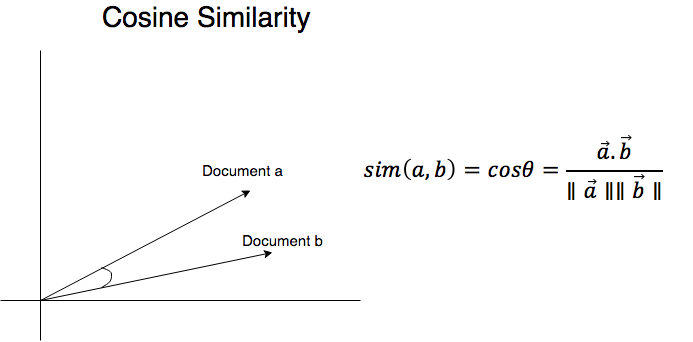by Ingroj Shrestha on Jan. 2, 2018This blog post is the second post in the series "Clustering Text Documents". In the previous blog post (Clustering Text Documents: TF-IDF Weighting), we represented a given set of documents as a vector of tf-idf weights and in this blog post we'll calculate the cosine of angles between those document vectors. But, before we jump into the calculations we'll discuss the basic concept of similarity and mathematical definition of cosine similarity.

Document similarity is the measure of how similar or alike two documents are. It is one of the central concepts in:

• Information retrieval

• Searching

• Document classification

• Clustering

• Recommendation systems

• ## Cosine Similarity: Basics

Cosine similarity is the measure of the cosine of angle between two vectors; in our case the two vectors are text documents, which are represented as vector of tf-idf weights. The cosine angle is the measure of overlap between the documents in terms of their content. We can find the cosine similarity between two documents by using the equation of dot product.

From the definition of the dot product we have,

$$\vec{a} . \vec{b} = ||\vec{a}|| ||\vec{b}|| cos\theta$$

Where,

• $\vec{a} = (a_1, a_2, ... a_n)$ and $\vec{b} = (b_1, b_2, ... b_n)$ two vectors with dimension $n$.

• $cos\theta$ is the angle between $\vec{a}$ and $\vec{b}$

• $||\vec{a}|| = \sqrt{a_1^2 + a_2^2 + ... + a_n^2}$ and $||\vec{b}|| = \sqrt{b_1^2 + b_2^2 + ... + b_n^2}$

• NOTE: $a_n$ and $b_n$ are the components of vectors $\vec{a}$ and $\vec{b}$, respectively. In our case they are the tf-idf values for each word in the given set of documents

By rearranging the equation we get,

$$cos\theta = \frac{\vec{a} . \vec{b}} {||\vec{a}|| ||\vec{b}||}$$

The value of cosine angle can usually be between -1 and 1. The angle between the documents vectors can only be less than or equal to $90^{\circ}$, which is why cosine similarity takes values between 0 and 1. The two documents are orthogonal when the angle between them is maximum i.e. $90^{\circ}$ while the documents are completely overlapping when the angle between them is $0^{\circ}$. So, lesser is the angle between two documents higher is the value of cosine similarity.## Cosine Similarity: Example

The document space we'll be using for the example is the same one we used in the previous blog post.

d1 = "त्यो घर रातो छ"
d2 = "यो निलो कलम हो"
d3 = "भाईको घर हो"


In the previous post, we transformed the above document space into the following tf-idf matrix.

$$M_{tf-idf} = \begin{bmatrix} 0.60534851 & 0.79596054 & 0 & 0 & 0 \\ 0 & 0 & 0.70710678 & 0.70710678 & 0 \\ 0.60534851 & 0 & 0 & 0 & 0.79596054 \\ \end{bmatrix}$$

Now, we'll calculate the cosine angle between document d1 and the other two documents (d2 and d3).

Cosine angle between d1 and d2 is,

$$cos\theta = \frac{d1 . d2}{||d1|| ||d2||}$$ $$= 0$$

Cosine angle between d1 and d3 is,

$$cos\theta = \frac{d1 . d3}{||d1|| ||d3||}$$ $$= 0.36644682$$

Similarly, the cosine angle between document d2 and d3 is,

$$cos\theta = \frac{d2 . d3}{||d2|| ||d3||}$$ $$= 0$$

Finally, we have the cosine similarity matrix for the given document space as follows:

$$M_{cosine-similarity} = \begin{bmatrix} 1 & 0 & 0.36644682 \\ 0 & 1 & 0 \\ 0.36644682 & 0 & 1 \\ \end{bmatrix}$$

Since, document d1 and d3 have one common word, while both the documents have nothing in common with document d2, the cosine similarity value for document d1 and d3 is better compared to that for document d1 and d2 or document d2 and d3. Hence, documents d1 and d3 are more similar to each other in terms of their content.

## Cosine Similarity: Implementation using Scikit-learn

We can use one of the two methods discussed in the previous blog post to transform the given document space into tf-idf matrix. We will be using the second method that uses TfidfVectorizer() class to transform document space into a tf-idf matrix.

from sklearn.feature_extraction.text import TfidfVectorizer
from stem.itrstem import IterativeStemmer
from tokenize.tokenizer import Tokenizer
from rmvstopwords import StopWordRemover

d1 = "त्यो घर रातो छ"
d2 = "यो निलो कलम हो"
d3 = "भाईको घर हो"
documents = [d1, d2, d3]

class StemmedTfidfVectorizer(TfidfVectorizer):
def build_analyzer(self):
analyzer = super(StemmedTfidfVectorizer, self).build_analyzer()
return lambda doc: (stemmer.stem(w) for w in analyzer(doc))

print(" ------------------- Method-II -------------------")
print("========= Vocabulary =========")
print(vectorizer.vocabulary_)
tfid_vectorizer = StemmedTfidfVectorizer(stop_words=StopWordRemover().get_stopwords(),
tokenizer=lambda text: Tokenizer().word_tokenize(text=text), analyzer='word')
tf_idf_matrix = tfid_vectorizer.fit_transform(documents)

print(tf_idf_matrix.todense())


OUTPUT:

------------------- Method-II -------------------
========= Vocabulary =========
{'घर': 0, 'रातो': 1, 'निलो':2, 'कलम': 3, 'भाई': 4}

[[ 0.60534851  0.79596054  0.          0.          0.        ]
[ 0.          0.          0.70710678  0.70710678   0.        ]
[ 0.60534851  0.          0.          0.           0.79596054]]



After transforming the document space into the tf-idf matrix, we can use the cosine_similarity() function to calculate cosine similarities between the given documents.

from sklearn.metrics.pairwise import cosine_similarity

------------------- Cosine Similarity -------------------
cosine_similarity = cosine_similarity(tf_idf_matrix)
print(cosine_similarity.todense())

OUTPUT:

------------------- Cosine Similarity -------------------
[[ 1.          0.          0.36644682]
[ 0.          1.           0.        ]
[ 0.36644682  0.           1.        ]]


## References

• Cosine Similarity - ML Wiki

• Machine Learning :: Cosine Similarity for Vector Space Models (Part III)

• Tags: Information Retrieval , Machine Learning